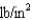# College Physics Reasoning and Relationships

Physics & Astronomy

## Quiz 10 :FluidsLooking for Introductory Physics Homework Help?

## Quiz 10 :Fluids

Showing 1 - 20 of 74The standard kilogram is a platinum-iridium cylinder 39.0 mm in height and 39.0 mm in diameter. What is the density of the material?
Free
Multiple Choice

AThe quantity "pressure" expressed in terms of the fundamental quantities (mass, length, time) is equivalent to:
Free
Multiple Choice

BThe pressure inside a commercial airliner is maintained at 1.00 atm (105 Pa). What is the net outward force exerted on a 1.0 m 2.0 m cabin door if the outside pressure is 0.40 atm?
Free
Multiple Choice

AA stonecutter's chisel has an edge area of 0.40 cm2. If the chisel is struck with a force of 45 N, what is the pressure exerted on the stone?
Multiple ChoiceThe Greenland ice sheet can be one km thick. Estimate the pressure underneath the ice where it is 0.80 km deep. (The density of ice is 918 kg/m3.)
Multiple ChoiceWhat is the total mass of the Earth's atmosphere? (The radius of the Earth is 6.4 106 m, and atmospheric pressure at the surface is 105 N/m2.)
Multiple ChoiceA solid object is made of two materials, one material having density of 2000 kg/m3 and the other having density of 8000 kg/m3. If the object contains equal volumes of the materials, what is its average density?
Multiple ChoiceA solid object is made of two materials, one material having density of 2000 kg/m3 and the other having density of 6000 kg/m3. If the object contains equal masses of the materials, what is its average density?
Multiple ChoiceA reading of a tire gauge tells you that the gauge pressure in a tire is 30. What is the absolute pressure in the tire? Assume that atmospheric pressure is 14.
Multiple ChoiceA container is filled with water and the pressure at the container bottom is P. If the container is instead filled with oil having a density of 0.80 that of water, what new bottom pressure results?
Multiple ChoiceA container is filled with water, and the pressure at the bottom of the container is P. Then the container is emptied halfway and topped off with oil of density 0.80 103 kg/m3, which floats on top of the water. What is the pressure at the bottom of the container now?
Multiple ChoiceAt a pressure of 1 atmosphere a column of mercury in a barometer is supported to the height h = 0.76 m. The density of mercury is 13.6 103 kg/m3. A barometer of similar design filled with water would support a column of water how high at a pressure of 1 atmosphere?
Multiple ChoiceWhat is the total force on the bottom of a filled 4.0-m diameter by 1.0-m deep round wading pool due to the weight of the air and the weight of the water? (Note that the pressure contribution from the atmosphere is 1.0 105 N/m2, the density of water is 1000 kg/m3, and g = 9.8 m/s2.)
Multiple ChoiceIn a large tank of liquid, the hydrostatic pressure at a given depth is a function of:
Multiple ChoiceBy what factor is the total pressure greater at a depth of 650 m in water than at the surface where the pressure is one atmosphere? (water density = 1.0 103 kg/m3, 1 atmosphere pressure = 1.01 105 N/m2, and g = 9.8 m/s2)
Multiple ChoiceIf the column of mercury in a barometer stands at 69.4 cm, what is the atmospheric pressure? (The density of mercury is 13.6 103 kg/m3 and g = 9.80 m/s2.)
Multiple ChoiceDams at two different locations are needed to form a lake. When the lake is filled, the water level will be at the top of both dams. Dam #2 is twice as high and three as wide as Dam #1. How much greater is the force of the water on Dam #2 than the force on Dam #1? (Ignore atmospheric pressure; it is pushing on both sides of the dams.)
Multiple ChoiceAtmospheric pressure is 1.0 105 N/m2, and the density of air is 1.29 kg/m3. If the density of air is constant as you get higher and higher, calculate the height of the atmosphere needed to produce this pressure.
Multiple Choice